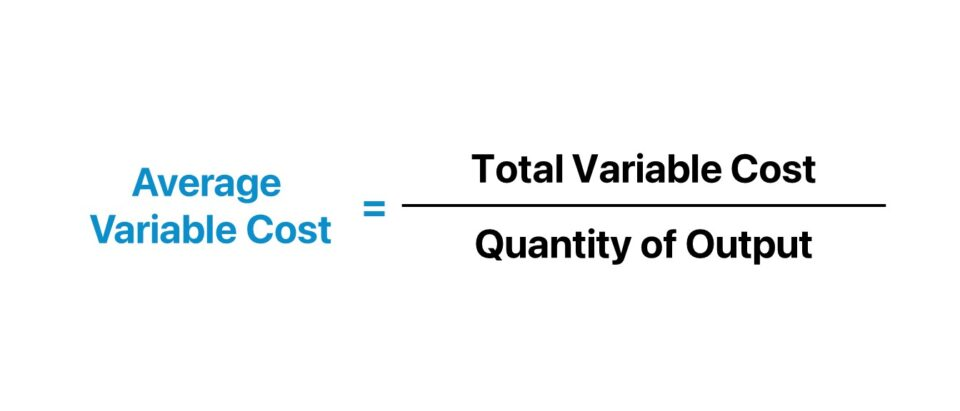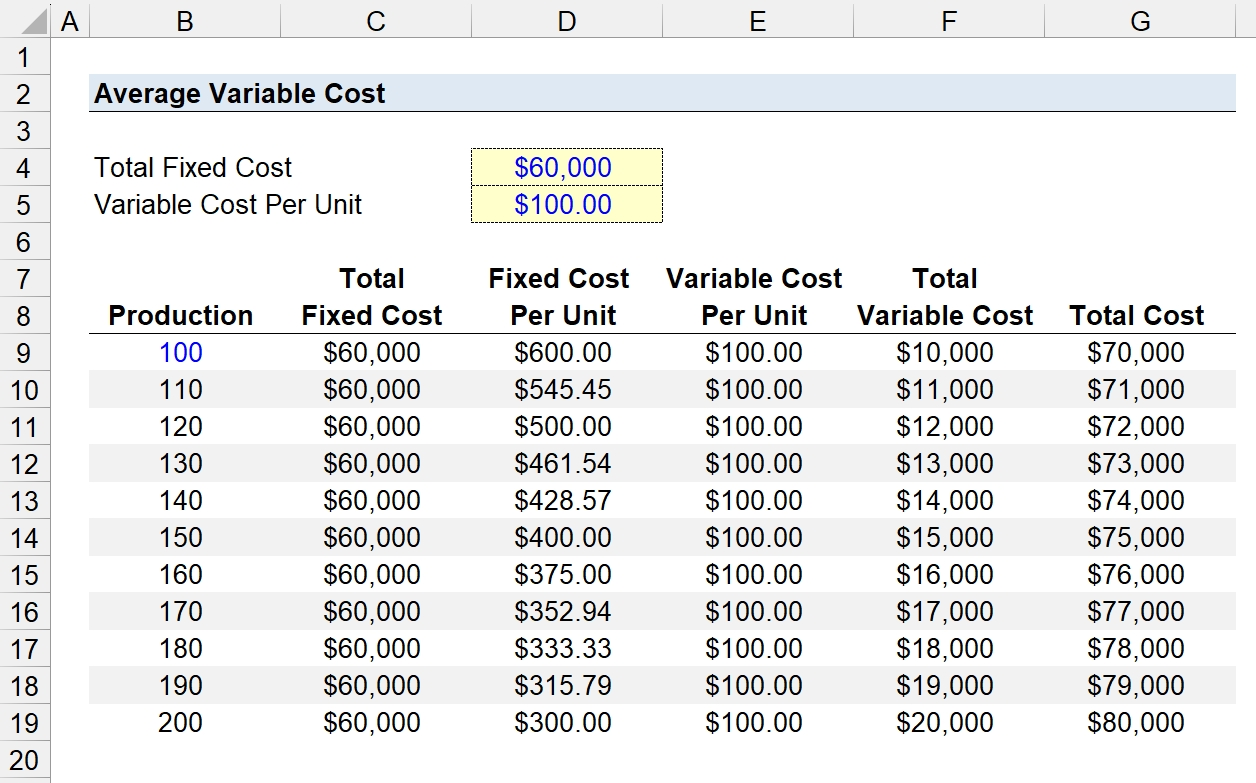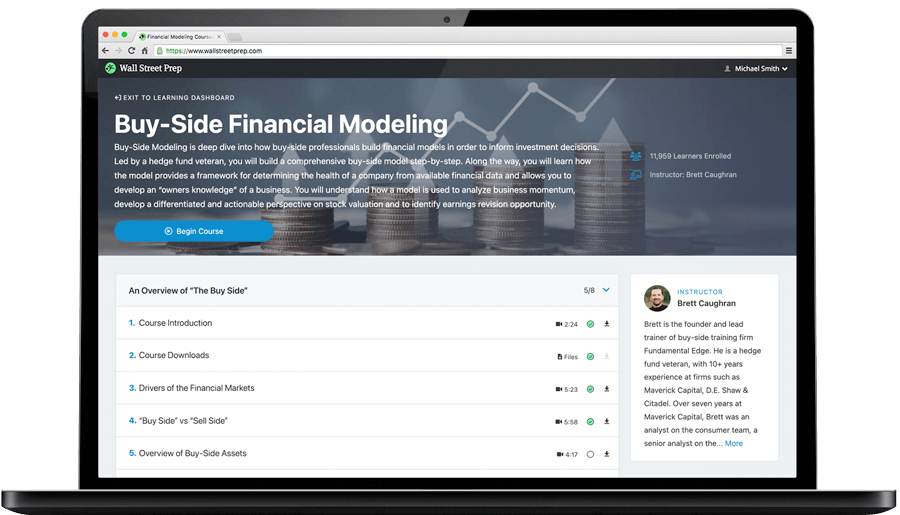Welcome to Wall Street Prep! Use code at checkout for 15% off.# Average Variable Cost (AVC)

Guide to Understanding Average Variable Cost (AVC)## How to Calculate Average Variable Cost

The average variable cost is an integral component in performing break-even analysis and estimating production capacity.

The average variable cost, often used interchangeably with the term “variable cost per unit,” measures the total variable cost incurred by a company relative to the quantity of output (i.e. production output).

By analyzing the average variable cost (AVC) metric, including its connection to the total cost (TC), management can optimize the company’s margin profile and improve its operating efficiency.

In practice, cost structure analysis refers to the disaggregation of a specific company’s cost composition into fixed costs and variable costs.

• Fixed Costs (FC) → Fixed costs remain constant under different levels of production output.
• Variable Costs (VC) → Variable costs fluctuate based on the levels of production output.

The following list contains the most common types of variable costs:

• Direct Labor
• Direct Material
• Sales Commission (and Bonuses)
• Shipping and Delivery Fees

Generally put, production should continue only if the monetary benefits received from customers (i.e. the price) exceed the fixed costs and variable costs.

Otherwise, the company must adjust its business model (e.g. increase pricing) because its profit margins will inevitably compress, which is not sustainable over the long run.

Quantifying the average variable cost, assuming the average fixed cost was determined beforehand, is enough to estimate a company’s break-even point (BEP).

The process of calculating the average variable cost (AVC) consists of three steps:

1. Identify the Variable Costs Embedded in Total Cost
2. Calculate the Sum of the Variable Costs
3. Divide the Total Variable Cost by the Production Output (Quantity)

## How to Analyze Average Variable Cost

Analyzing the variable cost per unit starts with grasping the internal and external factors that the company faces, which impacts its cost structure.

Understanding the cost structure of a company is necessary to set prices appropriately to ensure the break-even point is met (or exceeded, to generate a profit), as well as setting limitations on production capacity.

With that said, the cost structure concept is industry-specific, so companies operating in different industries cannot always be compared to each other.

• Capital-Intensive Industries (Asset-Heavy) → The cost structure of companies operating in capital-intensive industries, such as manufacturing and industrials, will have substantially more fixed costs, as the reliance on capital expenditures (Capex) is an inherent part of their business models.
• Service-Oriented Industries (Asset-Lite) → On the other hand, the cost structure of service-oriented industries like consulting and related professional services is composed mostly of variable costs, since labor is the most significant expense. For that reason, service-oriented industries tend to fare better in periods of economic contractions – not with regard to performance, but in terms of better avoiding financial distress and insolvency.
###### Cost Structure Analysis by Industry

To reiterate, the percent mix of fixed and variable costs in the cost structure of companies is contingent on the industry.

Certain manufacturing companies can continue to perform well amid periods of economic slowdown, especially if there are long-term B2B customer contracts, and the product is “mission-critical” to the end markets served (i.e. represent non-discretionary spending).

However, there is still significant risk for capital-intensive companies, especially if clients file for bankruptcy or there is substantial debt on the company’s balance sheet (i.e. high credit risk).

Conversely, labor-intensive industries can opt to reduce headcount to uphold margins and wait for the unfavorable economic conditions to pass, because such firms have more flexibility and levers to pull to ensure their “survival” (and service-oriented companies rarely carry much debt).

## Average Variable Cost Formula

The formula to calculate the average variable cost is as follows.

Average Variable Cost (AVC) = Total Variable Cost ÷ Production Output

Where:

• Total Variable Cost → The sum of all variable costs incurred by the company in a given period.
• Production Output → The total quantity of units produced by the company in the corresponding period.

## Average Variable Cost Calculator

We’ll now move to a modeling exercise, which you can access by filling out the form below.#### Get the Excel Template!Submitting...

## 1. Fixed Cost Calculation Example

Suppose we’re tasked with analyzing the average variable cost of an industrial manufacturer in 2023.

The manufacturer’s total fixed cost is \$60k, while the variable cost per unit is \$100.00.

• Total Fixed Cost = \$60,000
• Variable Cost Per Unit = \$100.00

To analyze the incremental change in the variable cost (and total cost) at various production levels, we’ll set up a table in Excel that ranges from 100 units to 200 units.

• Total Fixed Cost → The “Total Fixed Cost” column references the assumption cell formatted in blue font.
• Fixed Cost Per Unit → On the “Fixed Cost Per Unit” column, the total fixed cost is divided by the coinciding production quantity.

## 2. Average Variable Cost Calculation Example

With the fixed cost section of our table complete, our next step is to estimate the variable cost at various levels of production output.

The “Variable Cost Per Unit” column equals \$100 at all production levels, since the metric is expressed on a per-unit basis.

By multiplying the average variable cost by the quantity of units (or production), the total variable cost is \$10k.

• Total Variable Cost – Production @ 100 = \$100.00 × 100 = \$10,000## 2. Total Cost Per Unit Analysis Example

In the final part of our Excel exercise, we’ll estimate the total cost, given the two figures determined in the prior two steps.

Total Cost (TC) = Total Fixed Cost (FC) + Total Variable Cost (VC)

While the company’s total fixed cost per unit declines from the increase in production volume, the total variable cost per unit is constant.

However, the company’s total fixed cost – the gross value rather than per unit – is constant despite the increase in production volume, while the total variable cost rises in tandem.

In conclusion, the total variable cost increases from \$10k to \$20k at a production level of 100 and 200 units, respectively.

Hence, the total cost ranges between \$70k and \$80k in this scenario.Step-by-Step Online Course

### Learn Buy-Side (Hedge Fund) Modeling

Led by a former hedge fund PM (Maverick, Citadel, DE Shaw, Schonfeld), this program begins where financial modeling training ends — with a deep-dive into how buy-side analysts build financial models to make key investment decisions.